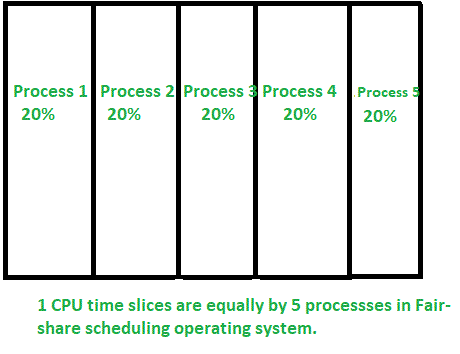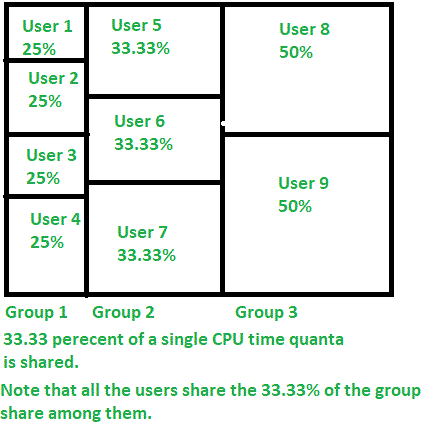# Fair-share CPU scheduling

• Difficulty Level : Easy
• Last Updated : 11 Oct, 2021

Fair-share scheduling is a scheduling algorithm that was first designed by Judy Kay and Piers Lauder at Sydney University in the 1980s. It is a scheduling algorithm for computer operating systems that dynamically distributes the time quanta “equally” to its users.

Time quantum is the processor time allowed for a process to run. But in a round-robin scheduling where the time slices or time quanta are allocated equally in a circular order, due to the distribution of time slices in a circular manner, any equal amount of time quanta will produce a similar output, therefore, an arbitrary distribution is required in this scenario.

Specificity of Fair-share scheduling :
This algorithm equally distributes the processor time to its users, for instance, there are 5 users (A, B, C, D, E)each of them are simultaneously executing a process, the scheduler divides the CPU periods such that all the users get the same share of the CPU cycles (100%/5) that is 20%. Even though, a user moves onto the second while the other at the first, the algorithm is so specific that it ensures that this user is attributed with only 10% for the second process making it a total of 20%.The scheduler logically divides an equal amount even though, another layer of partition is added, for example, if there were 3 groups present with different number of people in each group, the algorithm would still divide the same time for those groups, 100%/3= 33.33%, this 33.33% would be shared equally in the respective group depending on the number of users present in the group.To sum up, fair-share scheduling is an efficient strategy that creates a consistent user experience.

My Personal Notes arrow_drop_up